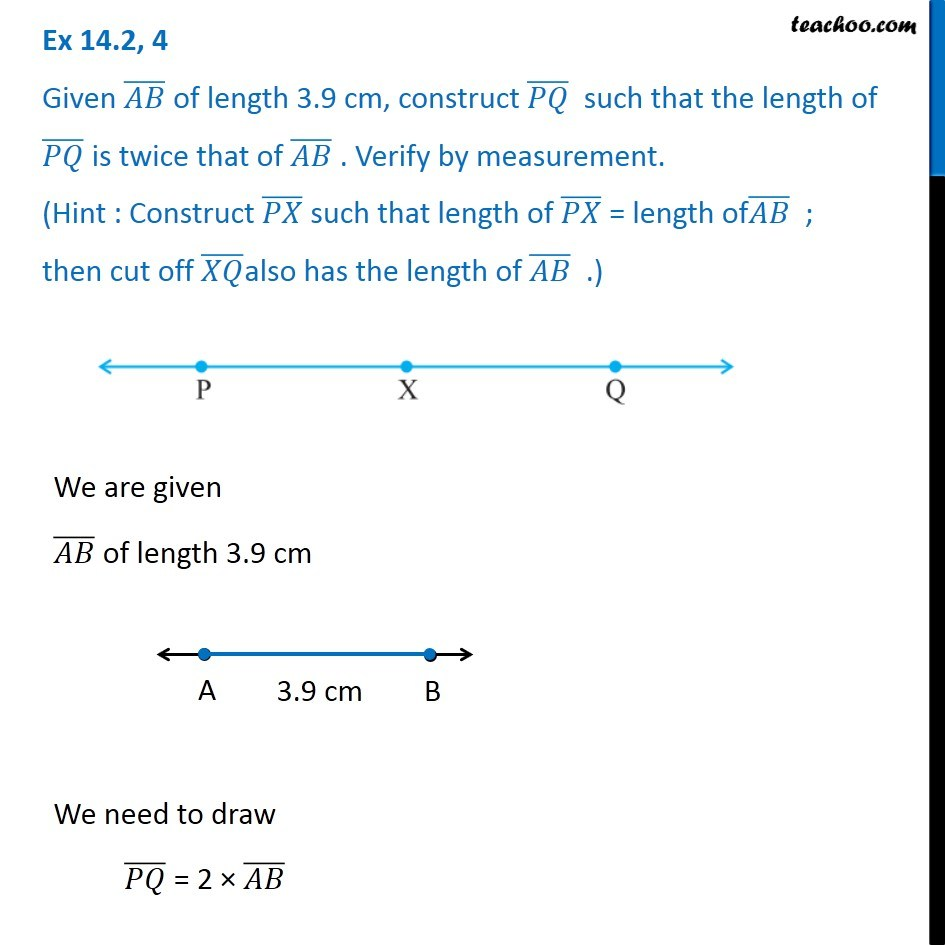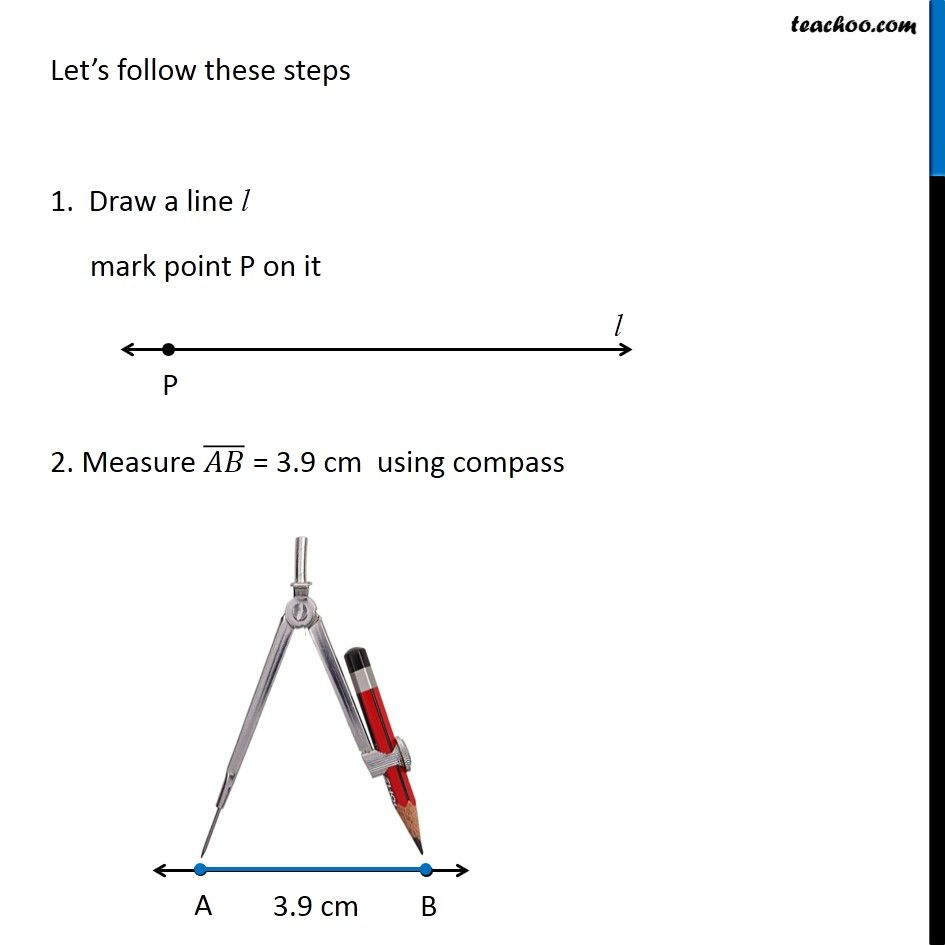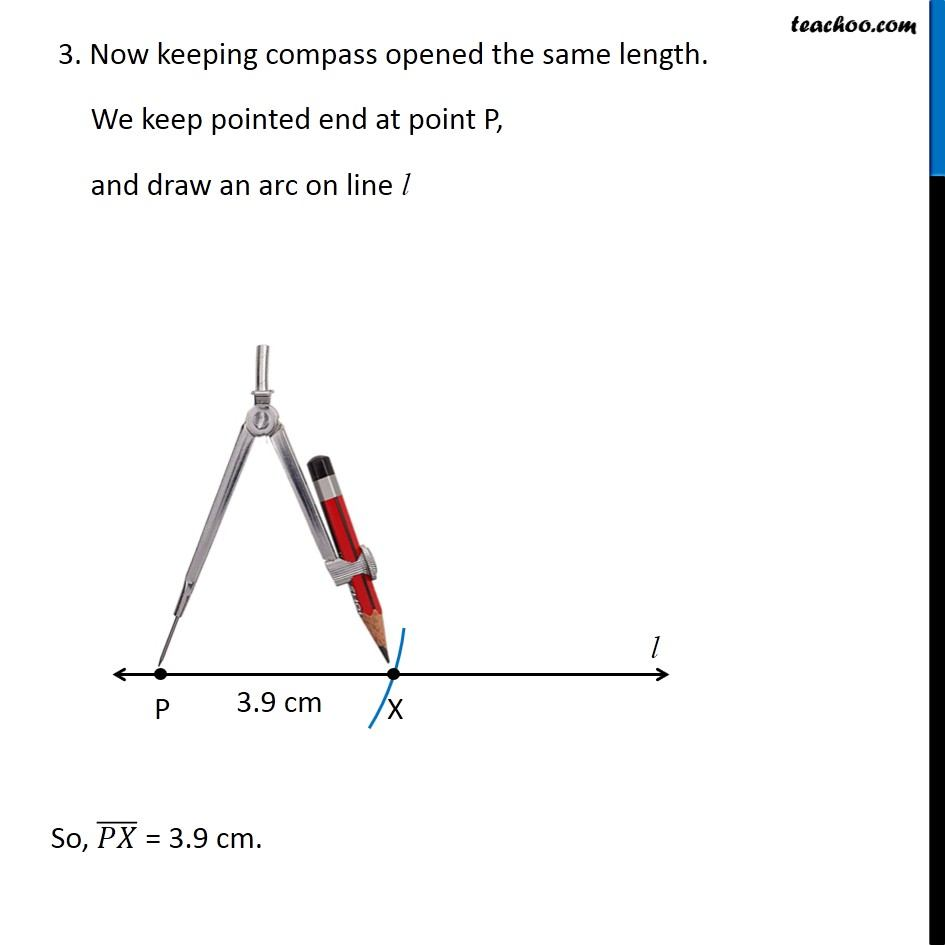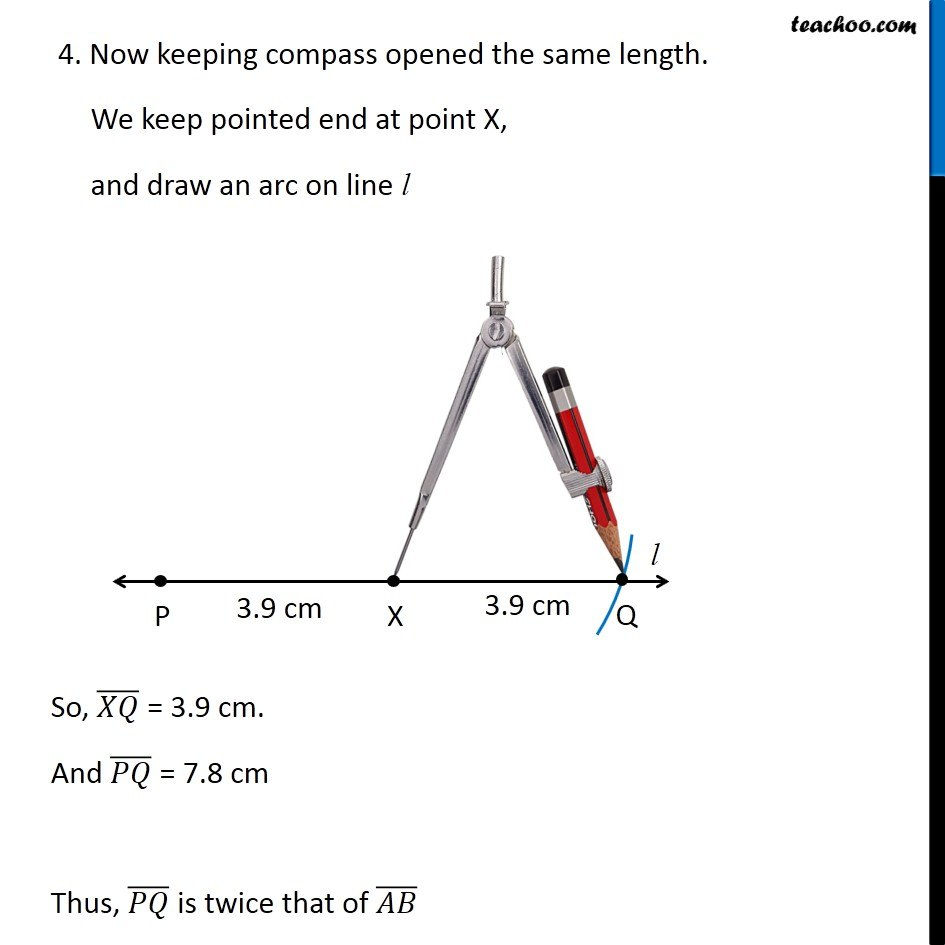Ex 14.2

Chapter 14 Class 6 Practical Geometry
Serial order wiseGet live Maths 1-on-1 Classs - Class 6 to 12

### Transcript

Ex 14.2, 4 Given (𝐴𝐵) ̅ of length 3.9 cm, construct (𝑃𝑄) ̅ such that the length of (𝑃𝑄) ̅ is twice that of (𝐴𝐵) ̅ . Verify by measurement. (Hint : Construct (𝑃𝑋) ̅ such that length of (𝑃𝑋) ̅ = length of(𝐴𝐵) ̅ ; then cut off (𝑋𝑄) ̅also has the length of (𝐴𝐵) ̅ .) We are given (𝐴𝐵) ̅ of length 3.9 cm We need to draw (𝑃𝑄) ̅ = 2 × (𝐴𝐵) ̅ Let’s follow these steps 1. Draw a line l mark point P on it 2. Measure (𝐴𝐵) ̅ = 3.9 cm using compass 3. Now keeping compass opened the same length. We keep pointed end at point P, and draw an arc on line l So, (𝑃𝑋) ̅ = 3.9 cm. 4. Now keeping compass opened the same length. We keep pointed end at point X, and draw an arc on line l So, (𝑋𝑄) ̅ = 3.9 cm. And (𝑃𝑄) ̅ = 7.8 cm Thus, (𝑃𝑄) ̅ is twice that of (𝐴𝐵) ̅